# Title 40

## SECTION 1036.535

### 1036.535 Determining steady-state engine fuel maps and fuel consumption at idle.

§ 1036.535 Determining steady-state engine fuel maps and fuel consumption at idle.

This section describes how to determine an engine's steady-state fuel map and fuel consumption at idle for model year 2021 and later vehicles. Vehicle manufacturers may need these values to demonstrate compliance with emission standards under 40 CFR part 1037 as described in § 1036.510.

(a) General test provisions. Perform fuel mapping using the procedure described in paragraph (b) of this section to establish measured fuel-consumption rates at a range of engine speed and load settings. Measure fuel consumption at idle using the procedure described in paragraph (c) of this section. If you perform cycle-average mapping for highway cruise cycles as described in § 1037.540, omit mapping under paragraph (b) of the section and instead perform mapping as described in paragraph (c) and (d) of this section. Use these measured fuel-consumption values to declare fuel-consumption rates for certification as described in paragraph (e) of this section.

(1) Map the engine as described in § 1036.510(a)(2) and (3), and perform emission measurements as described in 40 CFR 1065.501 and 1065.530 for discrete-mode steady-state testing. This section uses engine parameters and variables that are consistent with 40 CFR part 1065.

(2) Measure NOX emissions for each specified sampling period in g/s. You may perform these measurements using a NOX emission-measurement system that meets the requirements of 40 CFR part 1065, subpart J. Include these measured NOX values any time you report to us your fuel consumption values from testing under this section. If a system malfunction prevents you from measuring NOX emissions during a test under this section but the test otherwise gives valid results, you may consider this a valid test and omit the NOX emission measurements; however, we may require you to repeat the test if we determine that you inappropriately voided the test with respect to NOX emission measurement.

(b) Steady-state fuel mapping. Determine fuel-consumption rates for each engine configuration over a series of steady-state engine operating points as described in this paragraph (b). You may use shared data across an engine platform to the extent that the fuel-consumption rates remain valid. For example, if you test a high-output configuration and create a different configuration that uses the same fueling strategy but limits the engine operation to be a subset of that from the high-output configuration, you may use the fuel-consumption rates for the reduced number of mapped points for the low-output configuration, as long as the narrower map includes at least 70 points. Perform fuel mapping as follows:

(1) Select ten speed points that include warm idle speed, fnidle, the highest speed above maximum power at which 70% of maximum power occurs, nhi, and eight equally spaced points between fnidle and nhi. Control speed to within ±1% of nhi (see 40 CFR 1065.610(c)).

(2) Select ten torque values, including T = 0, maximum mapped torque, Tmax mapped, and eight equally spaced points between T = 0 and Tmax mapped. Replace any torque setpoints that are above the mapped torque at a given speed, Tmax, minus 5 percent of Tmax mapped. with one test point at Tmax. Control engine torque to within ±5% of Tmax mapped.

(3) You may need to adjust dynamometer settings any time the engine is operating on the low-speed or high-speed governor to maintain stable engine operation. You may change the dynamometer's speed setpoint as needed to avoid activating the engine's governor. You may alternatively set the dynamometer mode to torque-control, in which case speed can fall outside of ±1% of nhi.

(4) Precondition the engine as described in 40 CFR 1065.510(b)(2).

(5) Within 60 seconds after concluding the preconditioning procedure, operate the engine at nhi and Tmax.

(6) After the engine operates at the set speed and torque for 60 seconds, start recording measurements using one of the following methods:

(i) Carbon mass balance. Record speed and torque and measure emissions and other inputs needed to run the chemical balance in 40 CFR 1065.655(c) for (29 to 31) seconds; determine the corresponding mean values for the sampling period. We will use carbon mass balance.

(ii) Direct measurement of fuel flow. Record speed and torque and measure fuel consumption with a fuel flow meter for (29 to 31) seconds; determine the corresponding mean values for the sampling period.

(7) After completing the sampling period described in paragraph (b)(6) of this section, linearly ramp the engine over 15 seconds to the next lowest torque value while holding speed constant. Perform the measurements described at the new torque setting and repeat this sequence for all remaining torque values down to T = 0.

(8) Continue testing to complete fuel mapping as follows:

(i) At T = 0, linearly ramp the engine over 15 seconds to operate at the next lowest speed value and increase torque to Tmax. Perform measurements for all the torque values at the selected speed as described in paragraphs (b)(6) and (7) of this section. Repeat this sequence for all remaining speed values down to fnidle to complete the fuel-mapping procedure. You may interrupt the mapping sequence to calibrate emission-measurement instrumentation only during stabilization at Tmax for a given speed. If you use batch sampling to measure background emissions, you may sample periodically into the bag over the course of multiple test intervals defined by the period between calibrations of emission-measurement instrumentation. The background sample must be applied to correct emissions sampled over the test interval(s) between calibrations.

(ii) If an infrequent regeneration event occurs during fuel mapping, invalidate all the measurements made at that engine speed. Allow the regeneration event to finish, then restart engine stabilization at Tmax at the same engine speed and continue with measurements from that point in the fuel-mapping sequence.

(9) If you determine fuel-consumption rates using emission measurements from the raw or diluted exhaust, calculate the mean fuel mass flow rate, m fuel, for each point in the fuel map using the following equation: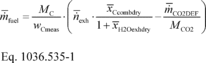Where: m fuel = mean fuel mass flow rate for a given fuel map setpoint, expressed to at least the nearest 0.001 g/s. MC = molar mass of carbon. wCmeas = carbon mass fraction of fuel (or mixture of test fuels) as determined in 40 CFR 1065.655(d), except that you may not use the default properties in Table 1 of 40 CFR 1065.655 to determine a, b, and wC for liquid fuels. n exh= the mean raw exhaust molar flow rate from which you measured emissions according to 40 CFR 1065.655. x Ccombdry= the mean concentration of carbon from fuel and any injected fluids in the exhaust per mole of dry exhaust as determined in 40 CFR 1065.655(c). x H2Oexhdry= the mean concentration of H2O in exhaust per mole of dry exhaust as determined in 40 CFR 1065.655(c). m CO2DEF= the mean CO2 mass emission rate resulting from diesel exhaust fluid decomposition as determined in paragraph (b)(10) of this section. If your engine does not use diesel exhaust fluid, or if you choose not to perform this correction, set m CO2DEF equal to 0. MCO2 = molar mass of carbon dioxide. Example: MC = 12.0107 g/mol wCmeas = 0.869 n exh= 25.534 mol/s x Ccombdry= 0.002805 mol/mol x H2Oexhdry= 0.0353 mol/mol m CO2DEF= 0.0726 g/s MCO2 = 44.0095 g/mol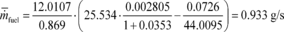(10) If you determine fuel-consumption rates using emission measurements with engines that utilize diesel exhaust fluid for NOX control, correct for the mean CO2 mass emissions resulting from diesel exhaust fluid decomposition at each fuel map setpoint using the following equation: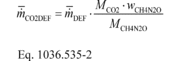Where: m DEF= the mean mass flow rate of injected urea solution diesel exhaust fluid for a given sampling period, determined directly from the engine control module, or measured separately, consistent with good engineering judgment. MCO2 = molar mass of carbon dioxide. wCH4N2O = mass fraction of urea in diesel exhaust fluid aqueous solution. Note that the subscript “CH4N2O” refers to urea as a pure compound and the subscript “DEF” refers to the aqueous 32.5% urea diesel exhaust fluid as a solution of urea in water with a nominal urea concentration of 32.5%. MCH4N2O = molar mass of urea. Example: m DEF= 0. 304 g/s MCO2 = 44.0095 g/mol wCH4N2O = 32.5% = 0.325 MCH4N2O = 60.05526 g/mol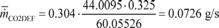(11) Correct the measured or calculated mean fuel mass flow rate, m fuel at each engine operating condition to a mass-specific net energy content of a reference fuel using the following equation: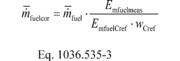Where: Emfuelmeas = the mass-specific net energy content of the test fuel as determined in § 1036.530(b)(1). EmfuelCref = the reference value of carbon-mass-specific net energy content for the appropriate fuel. Use the values shown in Table 1 of § 1036.530 for the designated fuel types, or values we approve for other fuel types. wCref = the reference value of carbon mass fraction for the test fuel as shown in Table 1 of § 1036.530 for the designated fuels. For other fuels, use the reference carbon mass fraction of diesel fuel for engines subject to compression-ignition standards, and use the reference carbon mass fraction of gasoline for engines subject to spark-ignition standards. Example: m fuel= 0.933 g/s Emfuelmeas = 42.7984 MJ/kgC EmfuelCref = 49.3112 MJ/kgC wCref = 0.874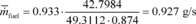(c) Fuel consumption at idle. Determine values for fuel-consumption rate at idle for each engine configuration as described in this paragraph (c). You may use shared data across engine configurations, consistent with good engineering judgment. Perform measurements as follows:

(1) Precondition the engine as described in 40 CFR 1065.510(b)(2).

(2) Within 60 seconds after concluding the preconditioning procedure, operate the engine at its minimum declared warm idle speed, fnidlemin, as described in 40 CFR 1065.510(b)(3), set zero torque, and start the sampling period. Continue sampling for (595 to 605) seconds. Perform measurements using carbon mass balance. Record speed and torque and measure emissions and other inputs as described in 40 CFR 1065.655(c); determine the corresponding mean values for the sampling period. Calculate the mean fuel mass flow rate, m fuel, during the sampling period as described in paragraph (b)(9) of this section.

Manufacturers may instead measure fuel consumption with a fuel flow meter and determine the corresponding mean values for the sampling period.

(3) Repeat the steps in paragraphs (c)(1) and (2) of this section with the engine set to operate at a torque setting of 100 N·m.

(4) Repeat the steps in paragraphs (c)(1) through (3) of this section with the engine operated at its declared maximum warm idle speed, fnidlemax.

(5) If an infrequent regeneration event occurs during this procedure, invalidate any measurements made at that idle condition. Allow the regeneration event to finish, then repeat the measurement and continue with the test sequence.

(6) Correct the measured or calculated mean fuel mass flow rate, m fuel at each of the four idle settings to account for mass-specific net energy content as described in paragraph (b)(11) of this section.

(d) Steady-state fuel maps used for cycle-average fuel mapping of the cruise cycles. Use the appropriate default steady-state engine fuel map as specified in Appendix I to this part to generate cycle-average fuel maps under § 1036.540, as amended based on the measurements specified in this paragraph (d). Measure fuel consumption at idle at the four specified engine operating conditions. For any values from the default map that lie within the boundaries of the engine speed and torque values represented by these idle-operating points, use the measured values instead of the default values. You may use shared data across engine configurations, consistent with good engineering judgment. Determine values for fuel-consumption rate at idle for each engine configuration as follows:

(1) Determine idle torque, Tidle, at the engine's maximum warm idle speed using the following equation: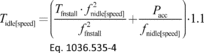Where: Tfnstall = the maximum engine torque at fnstall. fnidle[speed] = the applicable engine idle speed as described in this paragraph (d). fnstall = the stall speed of the torque converter; use fntest or 2250 rpm, whichever is lower.

Pacc = accessory power for the vehicle class; use 1500 W for Vocational Light HDV, 2500 W for Vocational Medium HDV, and 3500 W for Tractors and Vocational Heavy HDV.

Example: Tfnstall = 1870 N·m fntest = 1740.8 r/min = 182.30 rad/s fnstall = 1740.8 r/min = 182.30 rad/s fnidlemax = 700 r/min = 73.30 rad/s Pacc = 1500 W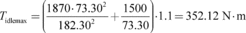(2) Precondition the engine as described in 40 CFR 1065.510(b)(2).

(3) Within 60 seconds after concluding the preconditioning procedure, operate the engine at its maximum declared warm idle speed, fnidlemax, as described in 40 CFR 1065.510(b)(3), set torque to the value determined in paragraph (d)(1) of this section, after the engine operates at the set speed and torque for 60 seconds, start the sampling period. Continue sampling for (29 to 31) seconds. Perform measurements using carbon mass balance. Record speed and torque and measure emissions and other inputs as described in 40 CFR 1065.655(c); determine the corresponding mean values for the sampling period. Calculate the mean fuel mass flow rate, m fuel, during the sampling period as described in paragraph (b)(9) of this section. Manufacturers may instead measure fuel consumption with a fuel flow meter and determine the corresponding mean values for the sampling period.

(4) Repeat the steps in paragraphs (d)(2) and (3) of this section with the engine set to operate at zero torque.

(5) Repeat the steps in paragraphs (d)(1) through (4) of this section with the engine operated at its declared minimum warm idle speed, fnidlemin.

(6) If an infrequent regeneration event occurs during this procedure, invalidate any measurements made at that idle condition. Allow the regeneration event to finish, then repeat the measurement and continue with the test sequence.

(7) Correct the measured or calculated mean fuel mass flow rate, m fuel at each of the four idle settings to account for mass-specific net energy content as described in paragraph (b)(11) of this section.

(e) Measured vs. declared fuel-consumption rates. Select fuel-consumption rates in g/s to characterize the engine's fuel maps. These declared values may not be lower than any corresponding measured values determined in paragraphs (b) through (d) of this section. You may select any value that is at or above the corresponding measured value. These declared fuel-consumption rates, which serve as emission standards under § 1036.108, are the values that vehicle manufacturers will use for certification under 40 CFR part 1037. Note that production engines are subject to GEM cycle-weighted limits as described in § 1036.301.